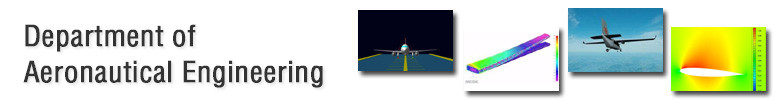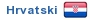Chair of Aircraft StructuresProject Laboratory
Research Projects
EducationStrength of Composite Aircraft Structures

 Semester Lectures + Exercises Subject Designation X 2 + 1 46495

Subject Holder:

Objective:

Introduction to strength analysis of basic elements of composite aeronautical structure in order to be able to size the typical structural elements of an aircraft

Description:

• Elasticity and stiffness matrices. Basic constitutive equations of layered materials. A, B, D matrices.
• Thermal and moisture induced stresses.
• Buckling of rectangular plates with material symmetry.
• Plates with circular and elliptical openings.
• Sandwich plates. Basic equations. Boundary conditions. Elasticity matrix.
• Bending of rectangular sandwich plates. Long plates. Influence of material model and layering setup.
• Buckling of rectangular sandwich plates. Wrinkling of face layers.
• Beams. Basic equations. Boundary conditions. Elasticity matrix and equivalent stiffness.
• Bending of thin-walled beams.
• Torsion of thin-walled beams.
• Buckling of shells.

Teaching material:

As provided to students during lectures